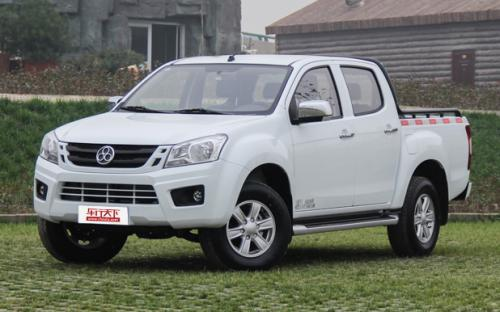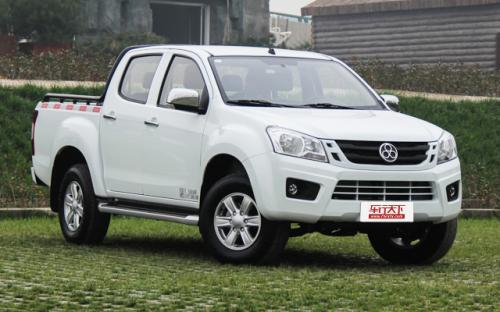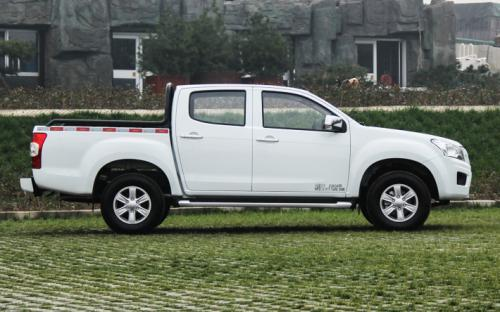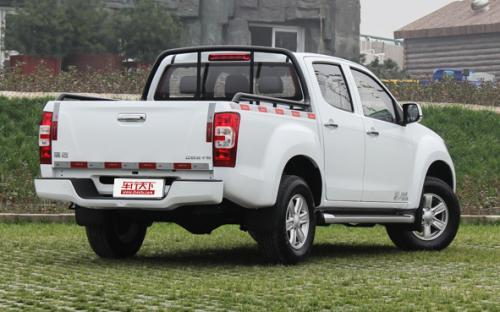## 江西五十铃 瑞迈0 种颜色可选2016款最低售价：8.48 万元起

5190×1860×1785(mm)(mm)(mm)##### 配置亮点：
• 胎压监测装置

• ISOFIX儿童座椅接口

• 车身稳定控制(ESC/ESP/DSC等)

• 电动天窗

• 定速巡航

• 后倒车雷达

• 真皮座椅

• GPS导航系统

• 氙气大灯

• 后视镜加热

2016款 2.8T两驱超豪华款JE493ZLQ4CA (88张)
• 2016款 2.8T两驱超豪华款JE493ZLQ4CA (88张)
• 2016款 2.8T两驱豪华款JE493ZLQ4CA (96张)江西五十铃 瑞迈 在售车型

排量 车型 厂商指导价 本地最低报价 购车工具
2.8L 2.8T两驱进取款JE493ZLQ4CA 柴油 5挡手动 8.48万 8.48万

询底价+对比
2.8T两驱豪华款JE493ZLQ4CA 柴油 5挡手动 8.88万 询底价+对比
2.8T两驱精英款JE493ZLQ4CA 柴油 5挡手动 9.18万 9.18万

询底价+对比
2.8T两驱超豪华款JE493ZLQ4CA 柴油 5挡手动 9.88万 询底价+对比
2.8T四驱豪华款JE493ZLQ4CA 柴油 5挡手动 10.68万 询底价+对比
2.8T四驱超豪华款JE493ZLQ4CA 柴油 5挡手动 11.68万 11.68万

询底价+对比

江西五十铃 瑞迈 经销商

查看更多 >>
更多 >>

本地降价排名

### 江西五十铃 瑞迈 动力加速

瑞迈 0-100公里加速时间分布在 0.0-秒 属于 超跑级

动力级别 加速时间 车型

江西五十铃 瑞迈 视频

江西五十铃 瑞迈 新闻资讯

# 江西五十铃瑞迈汽油版强势掀起订单热潮

行业动态 超过9060次关注

沐浴政策春风，柴油版瑞迈凭借强大的实用优势，一路高唱凯歌，连续刷新多个销售记录。而今，在汽油皮卡市场急需动能改造，排放升级的大环境下，汽油版瑞迈破世而出。

# 芯虔志承 瑞迈皮卡成就新春第一股订单热潮

新闻 超过9182次关注

瑞迈作为皮卡界的新锐，凭借五十铃在发动机、车身、底盘、装载以及性价比上的多重优势，继续沿续去年高歌猛进的势头，在行业内再起风云，掀起了新春第一股订单热潮。

# 江西五十铃瑞迈 瑞力勃发势不可挡

新闻 超过9641次关注

2017年是皮卡解禁政策试行的第二年，为了积极响应国家排放政策，也为了满足更多用户的需求，瑞迈将推出汽油版，在保持柴油市场涨势的同时，将在实用皮卡领域形成全...

# 瑞迈汽油低油耗与高动力挑T性能 一路芯动预售

评测 超过7583次关注

为了庆祝瑞迈皮卡在2016的辉煌表现、迎接汽油版瑞迈的芯动预售，瑞迈全系现推出全系钜惠。从2017年1月1日起，在活动期间消费者只要到店购买全系任何车型都将获得价...

# 瑞迈加长货箱上市 强力冲击轻卡及微面市场

行业动态 超过9624次关注

在今年的8月皮卡市场销量当中，整体销售数据迅速攀升，其中江西五十铃瑞迈车系的销量更是让行业看到皮卡的未来，随着瑞迈加长货箱的重磅上市，皮卡市场的销量有望取...

# 百年匠心厚载未来 江西五十铃瑞迈加长货箱预售开启

汽车导购 超过8841次关注

不知什么时候起，国内的汽车销售开始流行“加长版”，加个“L”以表明自己是加长版的身份。然而实际上，真正的加长版，远不是简单加个轴距那么简单，更要和车辆定位...

# 江西五十铃瑞迈的精品之道

行业动态 超过9230次关注

五十铃对于汽车爱好者来说是一个耳熟能详的品牌，作为国内生产该品牌汽车的江西五十铃一直也是在不断地探索、开拓新产品，瑞迈成为江西五十铃发展进程中不可忽视的...

猜你喜欢

﻿
• 快速找车
• 选择品牌
• 选择品牌
• A  奥迪
• A  阿斯顿·马丁
• A  阿尔法·罗密欧
• B  宝沃
• B  布加迪
• B  巴博斯
• B  保时捷
• B  宾利
• B  奔驰
• B  宝马
• B  本田
• B  别克
• B  标致
• B  比亚迪
• B  宝骏
• B  北汽制造
• B  北汽新能源
• B  北汽幻速
• B  北汽威旺
• B  北京汽车
• B  奔腾
• B  北汽绅宝
• C  长安
• C  长安商用
• C  长城
• C  昌河
• D  大众
• D  道奇
• D  DS
• D  东南
• D  东风风神
• D  东风风行
• D  东风小康
• D  东风风度
• D  东风
• F  福特
• F  丰田
• F  菲亚特
• F  法拉利
• F  福田
• F  福迪
• F  福汽启腾
• G  观致
• G  广汽传祺
• G  广汽吉奥
• G  GMC
• H  红旗
• H  汉腾汽车
• H  哈弗
• H  哈飞
• H  海格
• H  海马
• H  华颂
• H  黄海
• H  华泰
• H  恒天
• J  吉利汽车
• J  捷豹
• J  Jeep
• J  江淮
• J  江铃
• J  金杯
• J  九龙
• J  金旅
• K  凯翼
• K  凯迪拉克
• K  克莱斯勒
• K  科尼塞克
• K  卡威
• K  开瑞
• L  路虎
• L  林肯
• L  劳斯莱斯
• L  兰博基尼
• L  雷克萨斯
• L  铃木
• L  雷诺
• L  理念
• L  力帆
• L  莲花汽车
• L  猎豹
• L  路特斯
• L  陆风
• M  马自达
• M  MG
• M  MINI
• M  玛莎拉蒂
• M  摩根
• M  迈凯轮
• N  纳智捷
• O  欧宝
• O  讴歌
• O  欧朗
• Q  奇瑞
• Q  起亚
• Q  启辰
• R  日产
• R  荣威
• R  瑞麒
• S  三菱
• S  斯威汽车
• S  萨博
• S  smart
• S  斯柯达
• S  斯巴鲁
• S  思铭
• S  双龙
• S  上汽大通
• S  双环
• T  特斯拉
• T  腾势
• W  沃尔沃
• W  五菱汽车
• W  五十铃
• W  威兹曼
• W  威麟
• X  现代
• X  雪佛兰
• X  雪铁龙
• X  西雅特
• Y  一汽
• Y  英菲尼迪
• Y  英致
• Y  依维柯
• Y  野马汽车
• Y  永源
• Z  众泰
• Z  中华
• Z  中兴
• Z  知豆
• 选择车系
• 选择车系
• 车型对比
• 选择品牌
• 选择品牌
• A  奥迪
• A  阿斯顿·马丁
• A  阿尔法·罗密欧
• B  宝沃
• B  布加迪
• B  巴博斯
• B  保时捷
• B  宾利
• B  奔驰
• B  宝马
• B  本田
• B  别克
• B  标致
• B  比亚迪
• B  宝骏
• B  北汽制造
• B  北汽新能源
• B  北汽幻速
• B  北汽威旺
• B  北京汽车
• B  奔腾
• B  北汽绅宝
• C  长安
• C  长安商用
• C  长城
• C  昌河
• D  大众
• D  道奇
• D  DS
• D  东南
• D  东风风神
• D  东风风行
• D  东风小康
• D  东风风度
• D  东风
• F  福特
• F  丰田
• F  菲亚特
• F  法拉利
• F  福田
• F  福迪
• F  福汽启腾
• G  观致
• G  广汽传祺
• G  广汽吉奥
• G  GMC
• H  红旗
• H  汉腾汽车
• H  哈弗
• H  哈飞
• H  海格
• H  海马
• H  华颂
• H  黄海
• H  华泰
• H  恒天
• J  吉利汽车
• J  捷豹
• J  Jeep
• J  江淮
• J  江铃
• J  金杯
• J  九龙
• J  金旅
• K  凯翼
• K  凯迪拉克
• K  克莱斯勒
• K  科尼塞克
• K  卡威
• K  开瑞
• L  路虎
• L  林肯
• L  劳斯莱斯
• L  兰博基尼
• L  雷克萨斯
• L  铃木
• L  雷诺
• L  理念
• L  力帆
• L  莲花汽车
• L  猎豹
• L  路特斯
• L  陆风
• M  马自达
• M  MG
• M  MINI
• M  玛莎拉蒂
• M  摩根
• M  迈凯轮
• N  纳智捷
• O  欧宝
• O  讴歌
• O  欧朗
• Q  奇瑞
• Q  起亚
• Q  启辰
• R  日产
• R  荣威
• R  瑞麒
• S  三菱
• S  斯威汽车
• S  萨博
• S  smart
• S  斯柯达
• S  斯巴鲁
• S  思铭
• S  双龙
• S  上汽大通
• S  双环
• T  特斯拉
• T  腾势
• W  沃尔沃
• W  五菱汽车
• W  五十铃
• W  威兹曼
• W  威麟
• X  现代
• X  雪佛兰
• X  雪铁龙
• X  西雅特
• Y  一汽
• Y  英菲尼迪
• Y  英致
• Y  依维柯
• Y  野马汽车
• Y  永源
• Z  众泰
• Z  中华
• Z  中兴
• Z  知豆
• 选择车系
• 选择车系
• 选择车型
• 选择车型
• 意见反馈